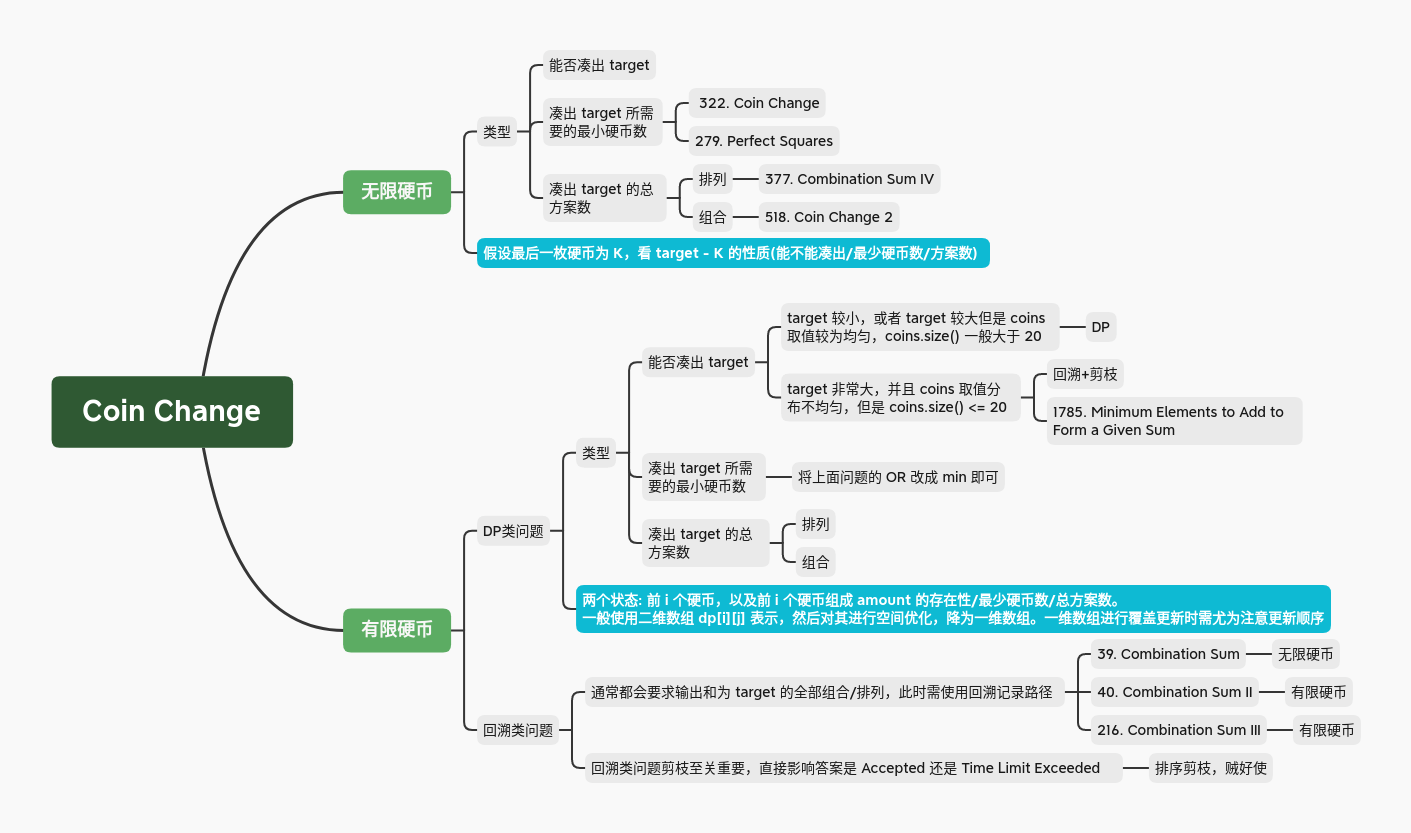Coin Change 是一道动态规划问题中一道非常经典的题目，这是因为只要对原有的 Coin Change 问题稍加修改，就可以得到更复杂的动态规划问题，甚至是回溯问题。

### 1. 无限硬币

#### 1.1 存在型动态规划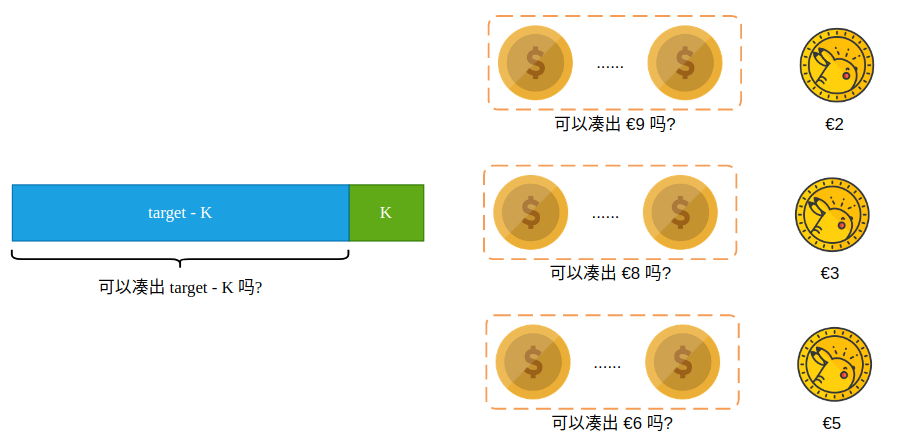``````bool solution(vector<int>& coins, int amount) {
vector<bool> dp(amount + 1, false);

// 当总金额为 0 时我们一个硬币都不选，所以为 true
dp = true;

// 计算金额为 1、2、3...amount 能否由 coins 拼出，sub 即为子金额
for (int sub = 1; sub <= amount; sub++) {
// 每一枚硬币都作为最后一枚硬币，看能不能拼出 sub - coin
for (int coin : coins) {
// 简单的来看，sub - coin 必须大于等于 0，才能作为数组下标
if (sub >= coin) {
dp[sub] = dp[sub] || dp[sub - coin];
}
}
}
return dp[amount];
}
``````

#### 1.2 最值型动态规划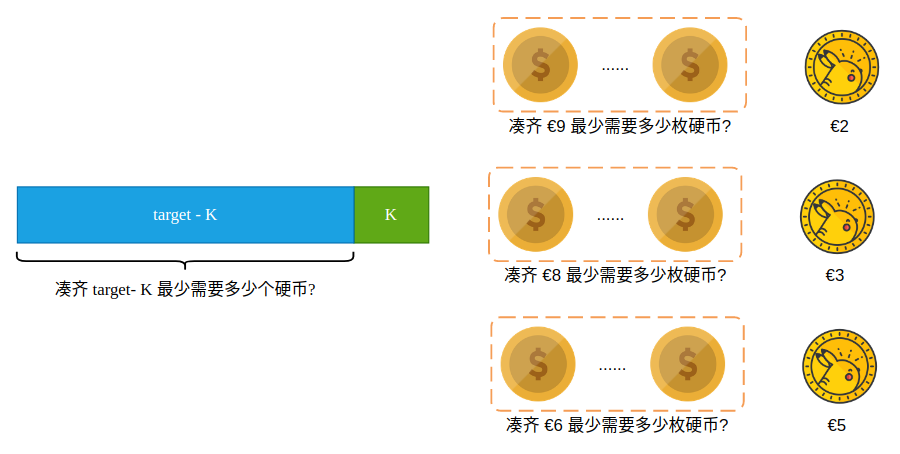``````int solution(vector<int>& coins, int amount) {
vector<int> dp(amount + 1, INT_MAX);

// 当金额为 0 时一枚硬币都不需要，所以 dp = 0
dp = 0;

for (int sub = 1; sub <= amount; sub++) {
for (int coin : coins) {
if (sub >= coin && dp[sub - coin] != INT_MAX) {
dp[sub] = min(dp[sub], dp[sub - coin] + 1);
}
}
}
return dp[amount] == INT_MAX ? -1 : dp[amount];
}
``````

#### 1.3 组合数型动态规划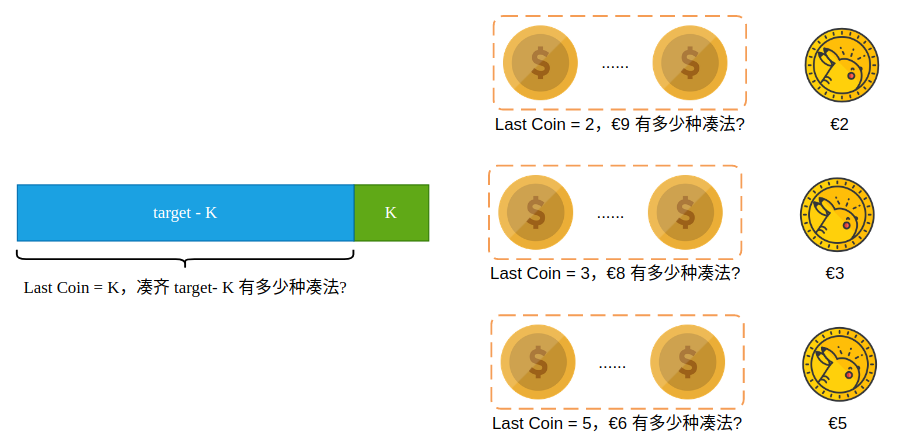``````int solution(vector<int>& coins, int amount) {
vector<int> dp(amount + 1, 0);

// 哪个硬币都不要，也是一种排列方式
dp = 1;

for (int sub = 1; sub <= amount; sub++) {
// 每一种不同面额的硬币作为 Last Coin 计算方案数
for (int coin : coins) {
if (sub >= coin)
dp[sub] += dp[sub - coin];
}
}
return dp[amount];
}
``````

LeetCode 中的 377. Combination Sum IV 其实就是一道求排列总方案的 Coin Change 问题。题干中并没有直接的使用 “permutation” 一词，而是在 Example 中说明了该题其实是一道排列型问题。

``````int solution(vector<int>& coins, int amount) {
vector<int> dp(amount + 1, 0);

// 同样的，哪个硬币都不选，也是一种组合方式
dp = 1;

for (int coin : coins){
for (int sub = 1; sub <= amount; sub++){
if (sub >= coin)
dp[sub] += dp[sub - coin];
}
}
return dp[amount];
}
``````

### 2. 有限硬币

#### 2.1 存在型动态规划

``````bool solution(vector<int>& coins, int amount) {
int n = coins.size();
vector<vector<bool>> dp(n + 1, vector<bool>(amount + 1, false));

// 前 0 枚硬币当然可以组成金额 0，因为一枚硬币都没有
dp = true;

for (int i = 1; i <= n; i++) {
for (int sub = 1; sub <= amount; sub++) {
// 前 i 枚硬币的最后一枚硬币为 i-1，面额为 coins[i-1]
int lastCoin = coins[i-1];
if (sub >= lastCoin)
dp[i][sub] = dp[i-1][sub] || dp[i-1][sub-lastCoin];
else
// 当最后一枚硬币的面值比金额还要大时候，我们肯定不能选择该枚硬币
dp[i][sub] = dp[i-1][sub];
}
}
return dp[n][amount];
}
``````

``````dp[i][sub] = dp[i-1][sub] || dp[i-1][sub-lastCoin];
``````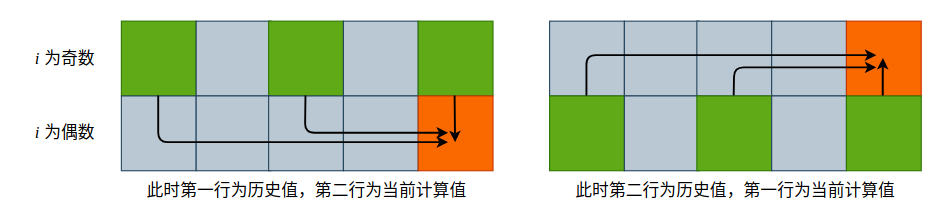``````bool solution(vector<int>& coins, int amount) {
int n = coins.size();
vector<vector<bool>> dp(2, vector<bool>(amount + 1, false));

dp = true;

for (int i = 1; i <= n; i++) {
for (int sub = 1; sub <= amount; sub++) {
int lastCoin = coins[i-1];
if (sub >= lastCoin)
dp[i%2][sub] = dp[(i-1)%2][sub] || dp[(i-1)%2][sub-lastCoin];
else
dp[i%2][sub] = dp[(i-1)%2][sub];
}
}
return dp[n%2][amount];
}
``````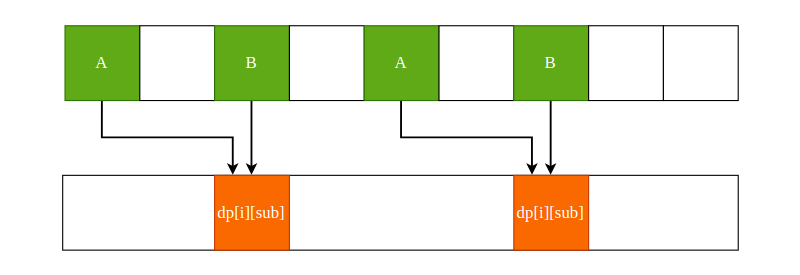``````bool solution(vector<int>& coins, int amount) {
int n = coins.size();
vector<bool> dp(amount + 1, false);

dp = true;

for (int i = 1; i <= n; i++) {
// 覆盖更新必须从右向左，因为左边儿的值还得用到呢
for (int sub = amount; sub >= 1; sub--) {
int lastCoin = coins[i-1];
if (sub >= lastCoin)
dp[sub] = dp[sub] || dp[sub-lastCoin];
}
}
return dp[amount];
}
``````

Leetcode 中 416. Partition Equal Subset Sum 就是一道有限硬币的存在型问题。只不过题目描述的比较隐晦，问一个数组能不能被分成两个元素和相等的子集，其实就是在问一个数组能不能找到一个子集，使其和为 sum(nums) / 2。

1780-Check-if-Number-is-a-Sum-of-Powers-of-Three.cpp

#### 2.2 最值型动态规划

``````dp[i][sub] = min(dp[i-1][sub], dp[i-1][sub-coins[i]] + 1);
``````

``````int solution(vector<int>& coins, int amount) {
int n = coins.size();
vector<int> dp(amount + 1, INT_MAX);

dp = 0;

for (int i = 1; i <= n; i++) {
for (int sub = amount; sub >= 1; sub--) {
int lastCoin = coins[i-1];
if (sub >= lastCoin && dp[sub-lastCoin] != INT_MAX)
dp[sub] = min(dp[sub], dp[sub-lastCoin] + 1);
}
}
return dp[amount] == INT_MAX ? -1 : dp[amount];
}
``````

### 3. 小结

Coin Change 类问题既可以看作是 0-1 背包问题，也可以看作是完全背包问题，不过他们的本质都是一样的: 对于第 K 个硬币/物品来说，在无限硬币中我们可以选择一个都不放和放多个，在单一使用硬币中我们可以选择放和不放，动态规划中一大类问题都可以使用 Coin Change 以及 0-1 背包问题解决。

Leetcode 上 Coin Change 类问题:

(1) 无限硬币

(2) 有限硬币